Publication date: 08/13/2020

## Time Series Basic Diagnostics Chart

The information that is shown in the Time Series Basic Diagnostics chart depends on the Time Series Report red triangle menu options. The red triangle menu options that show or hide information from the diagnostics chart are Autocorrelation, Partial Autocorrelation, Variogram, and AR Coefficients. By default, Autocorrelation and Partial Autocorrelation are shown.

### Autocorrelation Chart

The Autocorrelation option shows or hides the following columns in the Time Series Basic Diagnostics chart:

Lag

The number of periods between points.

Note: The number of lags begins with 0 as the default. To compute correlations beginning with lag 1, modify the JMP preferences before generating the graph. Select File > Preferences > Platforms > Time Series, and then select Suppress Lag 0 in ACF and PACF.

AutoCorr

The autocorrelation for the kth lag, computed as follows: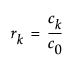where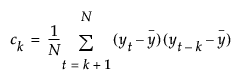and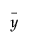is the mean of the N non-missing points in the time series. By definition, the first autocorrelation (lag 0) always has length 1.

The bars graphically depict the autocorrelations. The blue curves represent twice the large-lag standard error (± 2 standard errors), computed as follows: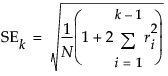Ljung-Box Q

Used to test whether a group of autocorrelations is significantly different from zero, or to test that the residuals from a model can be distinguished from white noise. Q is the test statistic.

p-Value

The p-value from the Ljung-Box test.

### Partial Autocorrelation Chart

The Partial Autocorrelation option shows or hides the following columns in the Time Series Basic Diagnostics chart:

Lag

The number of periods between points.

Partial

The partial autocorrelation for the kth lag.

The bars graphically depict the partial autocorrelations. The blue lines represent ± 2 standard errors for approximate 95% prediction limits, where the standard error is computed as follows: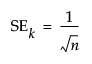for all k

### Variogram Chart

The Variogram option shows or hides the following columns in the Time Series Basic Diagnostics chart:

Lag

The number of periods between points.

Variogram

The variogram measures the variance of the differences of points k lags apart and compares it to that for points one lag apart. The variogram is computed from the autocorrelations as follows: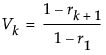where rk is the autocorrelation at lag k.

### AR Coefficients Chart

The AR Coefficients option shows or hides the following columns in the Time Series Basic Diagnostics chart:

Lag

The number of periods between points.

AR Coef

The coefficients approximate those that you would obtain from fitting a high-order, purely autoregressive model.